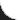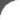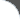Home | Tutorials | Articles | Videos | Products | Tools | Search
 Interviews | Open Source | Tag Cloud | Follow Us | Bookmark | ContactIn Browser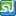StumbleUpon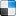del.icio.us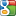Google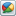Google Buzz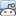reddit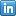LinkedIn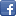Facebook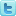TwitterLinkedin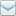E-Mail
 Java > Collection > Enum > Enum Instance Methods

# Enum Instance Methods

The following example shows how to implement instance specific methods with enum. All enum types implicitly implements java.io.Serializable and java.lang.Comparable. Enum provides the following APIs,

```public final String name()
- Returns name of enum constant.
```
We can see that constructor is accepting shape area description as string argument and provides an abstract method for enum constants to implement. Each enum constant provides its own implementation of 'getArea' method.
```public abstract double getArea(int radius)
```

 File Name  : com/bethecoder/tutorials/utils/enums/EnumInstanceMethods.java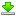Author  : Sudhakar KV Email  : [email protected]

 ``` package com.bethecoder.tutorials.utils.enums; enum Shape {   CIRCLE("Area of Circle") {     @Override     public double getArea(int radius) {       //pi*r^2       return Math.PI * Math.pow(radius, 2);     }   },      SQUARE("Area of Square") {     @Override     public double getArea(int radius) {       //length^2       return Math.pow(radius, 2);     }   };   /**    * Instance method to be overridden by each     * enum constant.    *     * @param radius    * @return    */   public abstract double getArea(int radius);      private String shapeName;   Shape(String shapeName) {     this.shapeName = shapeName;   }   public String getShapeName() {     return shapeName;   }   public void setShapeName(String shapeName) {     this.shapeName = shapeName;   }      public String toString() {     return getShapeName() + "[" + name() + "]";   } } public class EnumInstanceMethods {   /**    * @param args    */   public static void main(String[] args) {     int radius = 0;     System.out.println(Shape.SQUARE + "\t\t" + Shape.CIRCLE);          for (int i = 0 ; i < 4 ; i ++) {       radius = (i+1) * 10;       System.out.print(Shape.SQUARE.getArea(radius));              System.out.print("\t\t\t\t");       System.out.print(Shape.CIRCLE.getArea(radius));       System.out.println();     }   }    }```

It gives the following output,
```Area of Square[SQUARE]		Area of Circle[CIRCLE]
100.0				314.1592653589793
400.0				1256.6370614359173
900.0				2827.4333882308138
1600.0				5026.548245743669
```